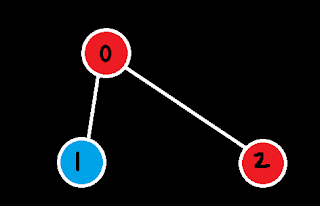Given an adjacency list of a graph adj  of V no. of vertices having 0 based index. Check whether the graph is bipartite or not.

graph is a bipartite if it can be coloured in two ways

Example 1:

```input:
0 is connected to 1 and 2
1 is connected to 0
2 is connected to 0

Output: 1
Explanation: The given graph can be colored
in two colors so, it is a bipartite graph.
```

Example 2:

```Input:0 is connected to 2 and 3
1 is connected to 3
2 is connected to 0 and 3
3 is connected to 0, 1 and 2

Output: 0

```

c++ implementation:

```// { Driver Code Starts
#include<bits/stdc++.h>
using namespace std;

// } Driver Code Ends
class Solution {
public:

//the main bipartite program starts here
bool dfs(int n,vector<int>a[],int vis[],int col[],int x,int c)
{
col[x]=c;

for(auto y:a[x])
{
if(col[y]==-1)
{
if(dfs(n,a,vis,col,y,!c)==0)
return 0;
}
else
{
if(col[x]==col[y])
return 0;
}
}
return 1;
}
bool isBipartite(int n, vector<int>a[]){
int vis[n];int col[n];

for(int i=0;i<n;i++)
{
vis[i]=0;
col[i]=-1;
}

for(int i=0;i<n;i++)
{
if(col[i]==-1)
{
if(dfs(n,a,vis,col,i,0)==0)
return 0;
}

}
return 1;

}
//------------
};

// { Driver Code Starts.
int main(){
int tc;
cin >> tc;
while(tc--){
int V, E;
cin >> V >> E;
for(int i = 0; i < E; i++){
int u, v;
cin >> u >> v;
}
Solution obj;